# 事件触发下混合时滞神经网络的状态估计*

(重庆交通大学 数学与统计学院, 重庆 400074)

(我刊编委宋乾坤来稿)

## 引 言

1) 首次研究了具有混合时滞的神经网络基于事件触发机制的状态估计问题；

2) 引入了一个具有指数衰减结构的新型事件触发机制，既能有效降低信息更新频率又能确保误差系统指数稳定

## 1 预 备 知 识

### 1.1 模型描述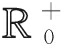和N分别表示非负实数和非负整数集;Rn和Rn×m分别表示n维欧氏空间和n×m维实矩阵的集合;|x|和xT分别表示向量x的模和它的转置; 对于A∈Rn×nλmax(A)和λmin(A)分别表示矩阵A的最大特征值和最小特征值，表示矩阵的范数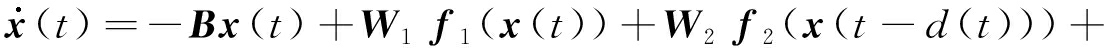W3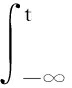K(t-s)f3(x(s))ds+J

(1)

y(t)=Cx(t)，

(2)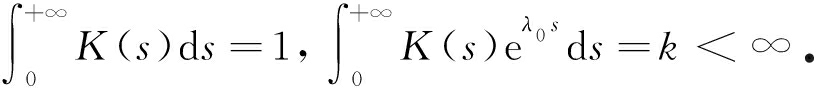|fi(x)-fi(y)|≤|Li(x-y)|， i=1,2,3，

(3)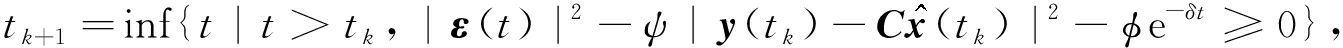(4)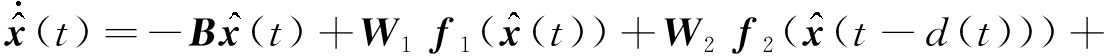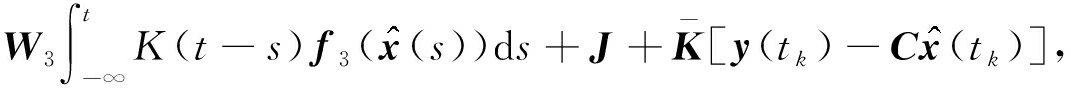(5)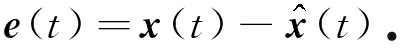(6)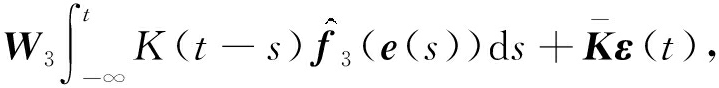(7)

### 1.2 基本定义和引理

|e(t)|2Me-λt

2xTyαxTx+α-1yTy

λmin(P-1Q)xTPxxTQxλmax(P-1Q)xTPx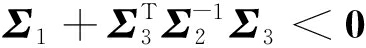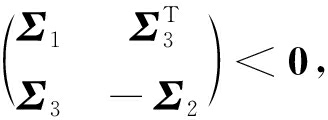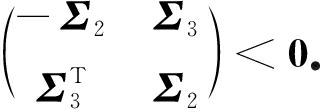## 2 主 要 结 果

Π+μP<0,

(8)

-μ+ζ1+γ+ω<0,

(9)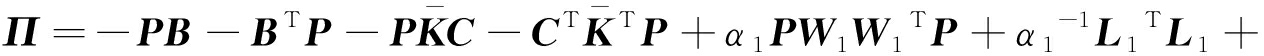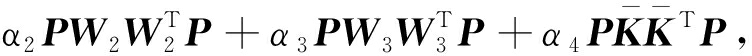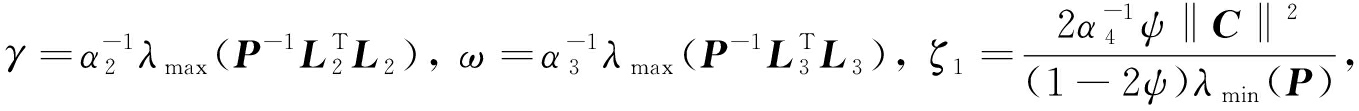V(t)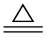V(e(t))=eT(t)Pe(t)

(10)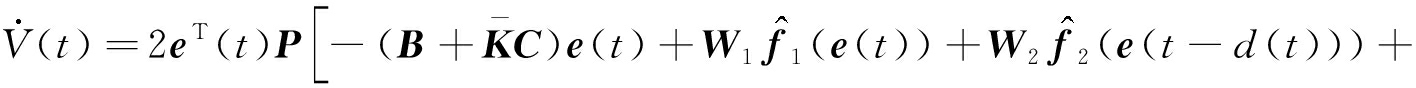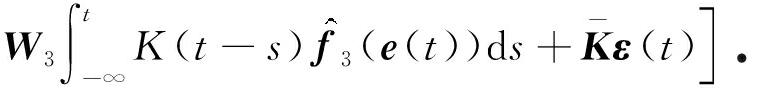(11)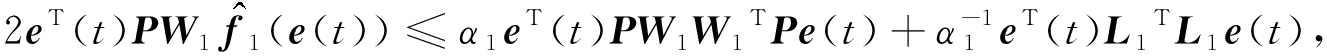(12)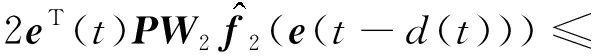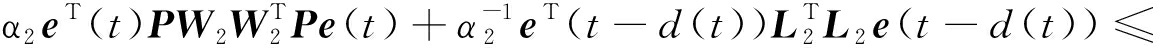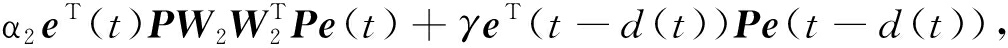(13)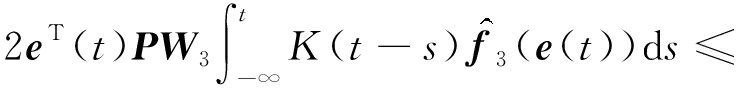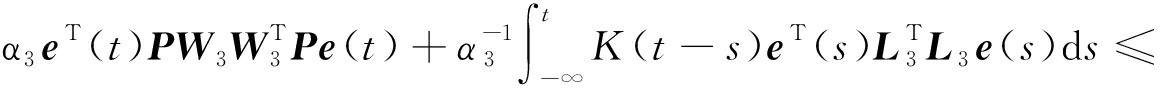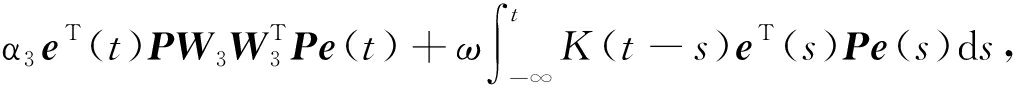(14)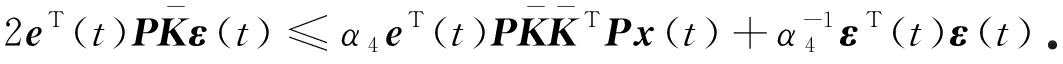(15)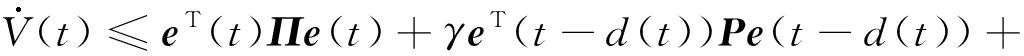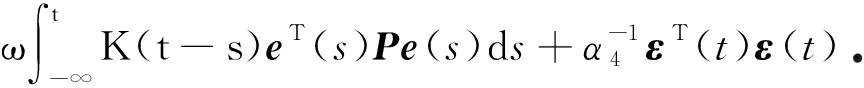(16)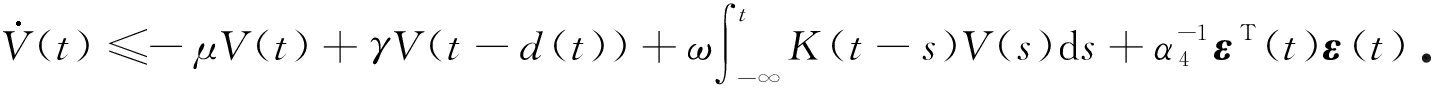(17)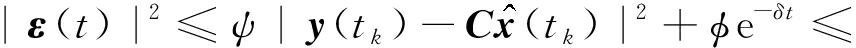2ψC2|e(t)|2+2ψ|ε(t)|2+φe-δt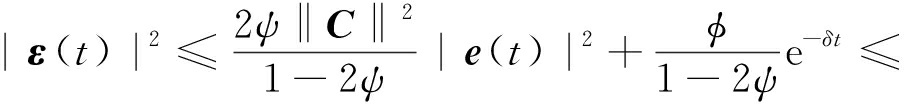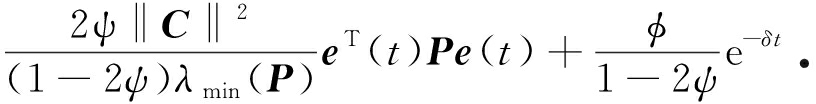(18)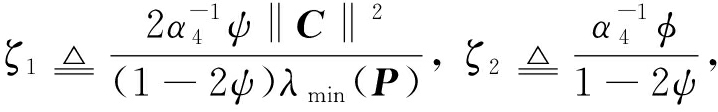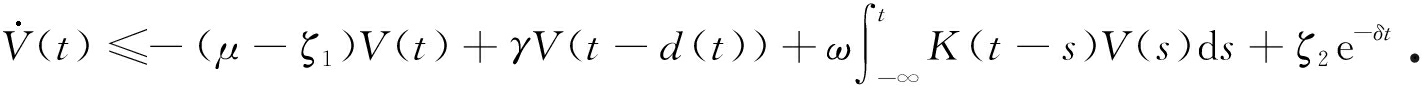(19)

V(t)=V(0)e-(μ-ζ1)t+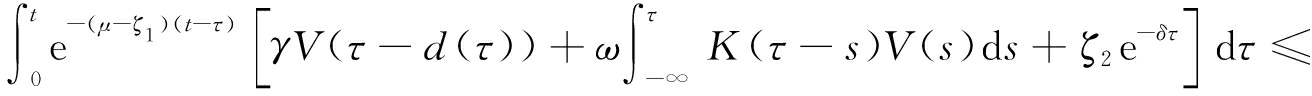θ‖e-(μ-ζ1)t+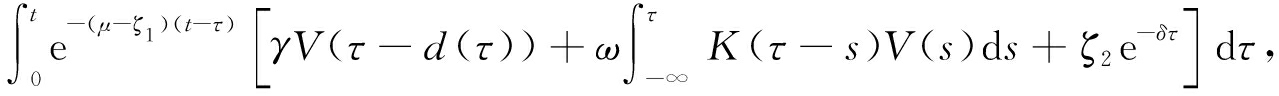(20)

V(t)≤Ze-δt

(21)

λ-μ+ζ1+γeλd+ω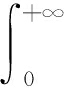K(s)eλsds=0，

(22)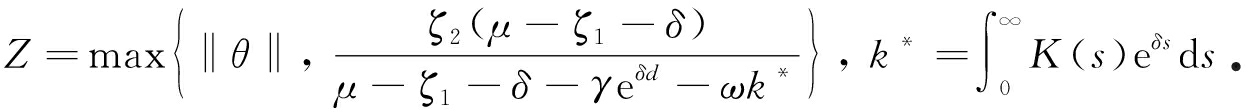V(t)≤‖θ‖≤ZZe-δt

(23)

V(t)>Ze-δt

(24)

t*inf{t>0|V(t)>Ze-δt}根据V(t)的连续性，我们可知

V(t)<Ze-δt, t<t*

(25)

V(t*)=Ze-δt*

(26)

V(t*)≤‖θ‖e-(μ-ζ1)t*+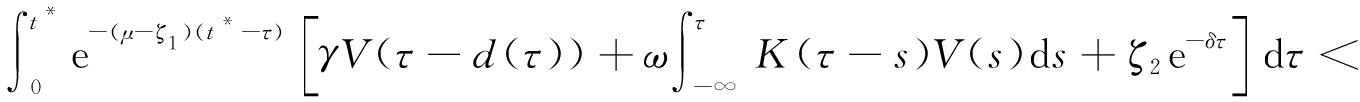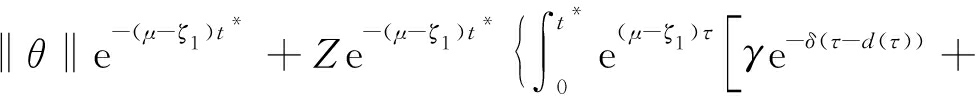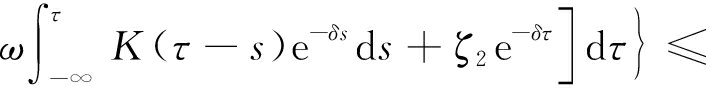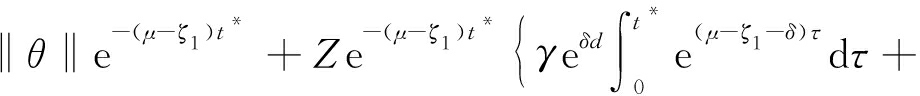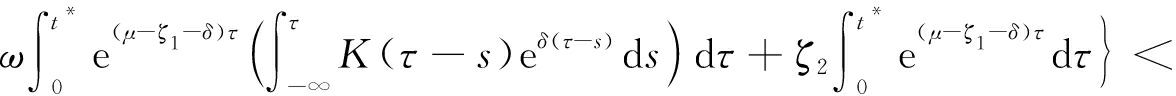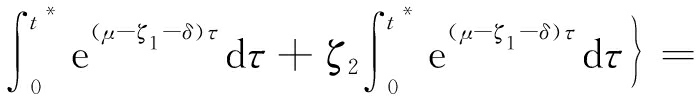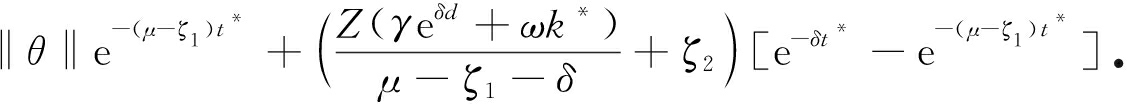(27)

V(t*)<‖θ‖e-δt*=Ze-δt*

(28)

θ‖-Z(γeδd+ωk*)/(μ-ζ1-δ)-ζ2≤0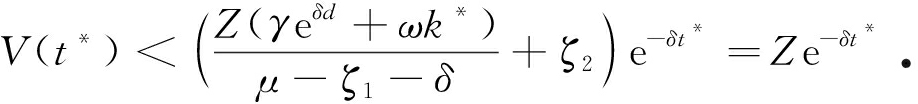(29)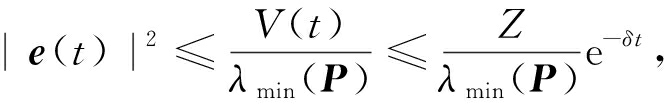(30)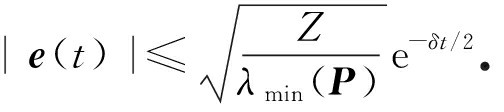(31)W2‖‖L2‖|e(t-d(t))|+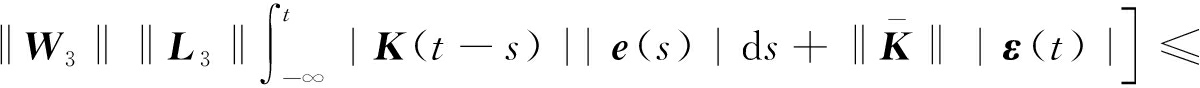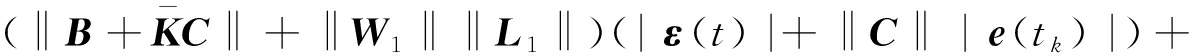C‖‖W2‖‖L2‖|e(t-d)|+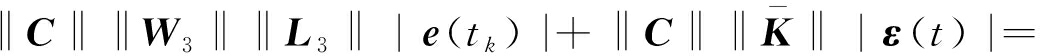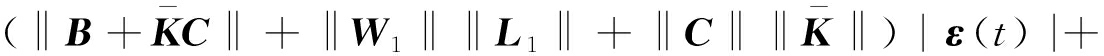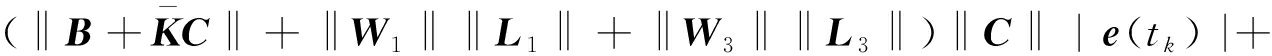C‖‖W2‖‖L2‖|e(t-d)|，

(32)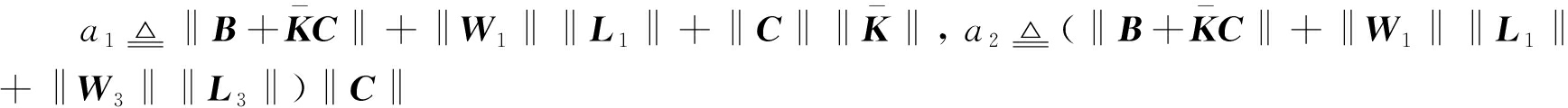以及a3C‖‖W2‖‖L2‖，可以得到

D+|ε(t)| ≤a1|ε(t)|+a2|e(tk)|+a3|e(t-d)|

(33)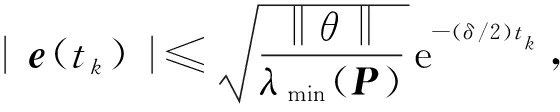(34)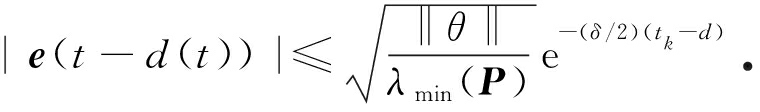(35)

D+|ε(t)|≤a1|ε(t)|+a2βe-(δ/2)tk+a3βe-(δ/2)(tk-d)a1|ε(t)|+M

(36)

e-a1(t-tk)D+|ε(t)|≤a1e-a1(t-tk)|ε(t)|+Me-a1(t-tk),

D+(e-a1(t-tk)|ε(t)|)≤Me-a1(t-tk)

(37)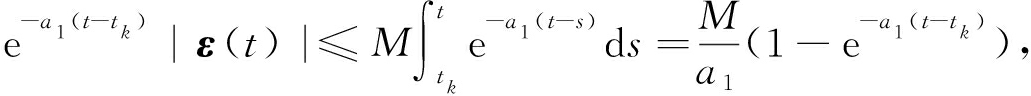(38)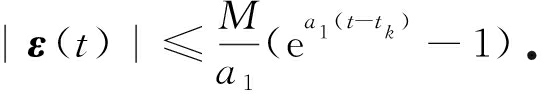(39)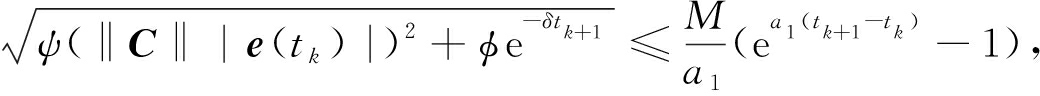(40)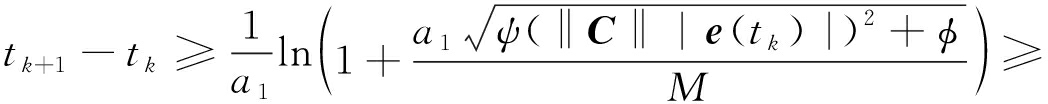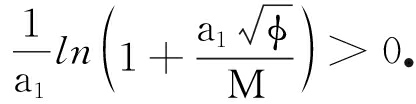(41)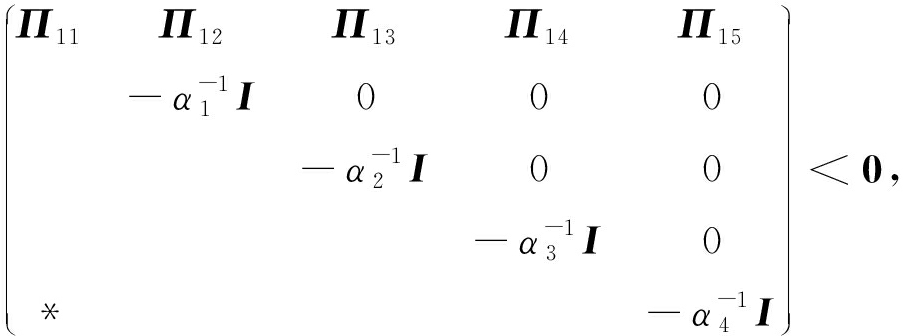(42)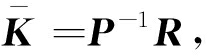(43)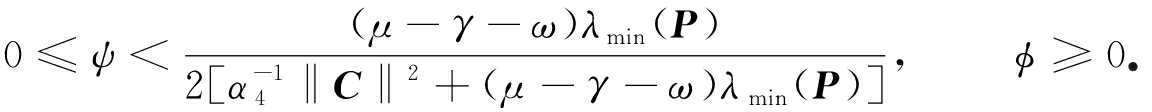(44)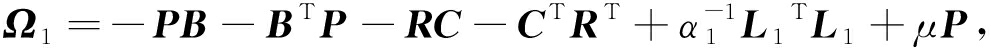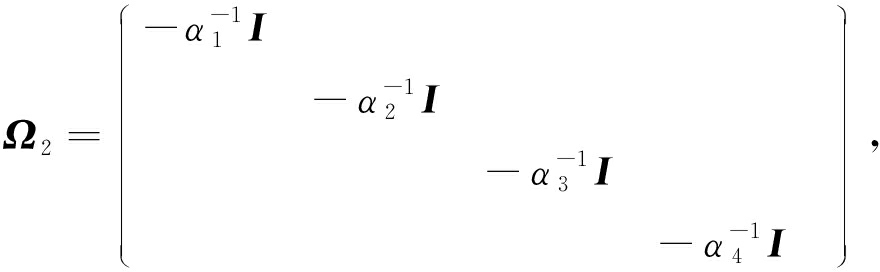Ω3=(PW1 PW2 PW3 R)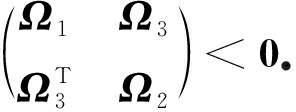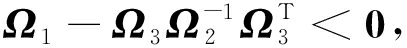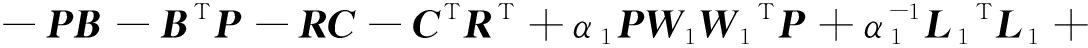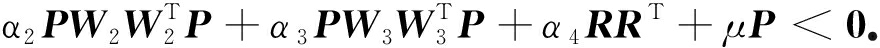## 3 例子与仿真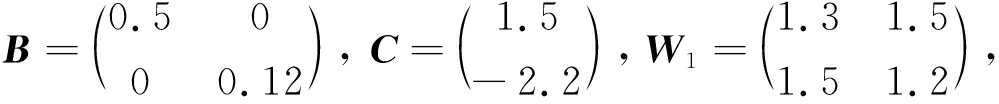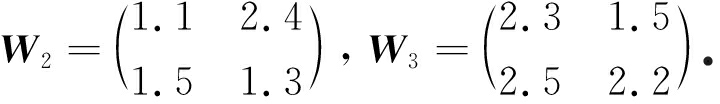μ=2.1，利用MATLAB工具箱求解不等式(42)～(44)，可得如下可行解：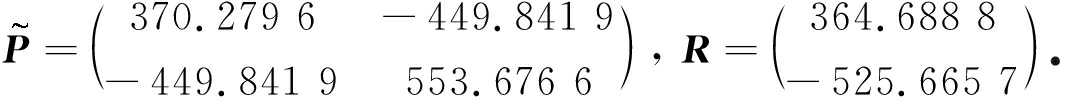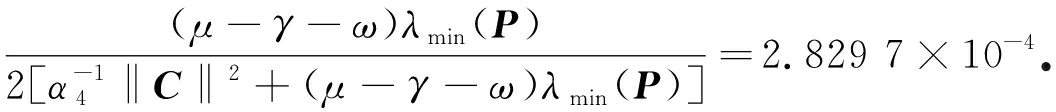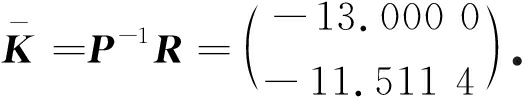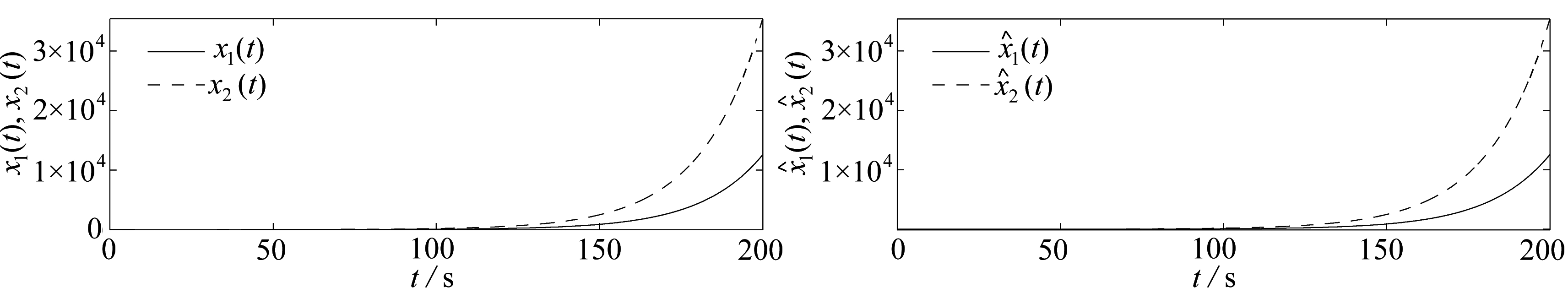(a) 神经网络状态 (b) 估计器状态
(a) The state of the neural network(b) The state of the estimator

Fig. 1 The states of the neural network and the estimator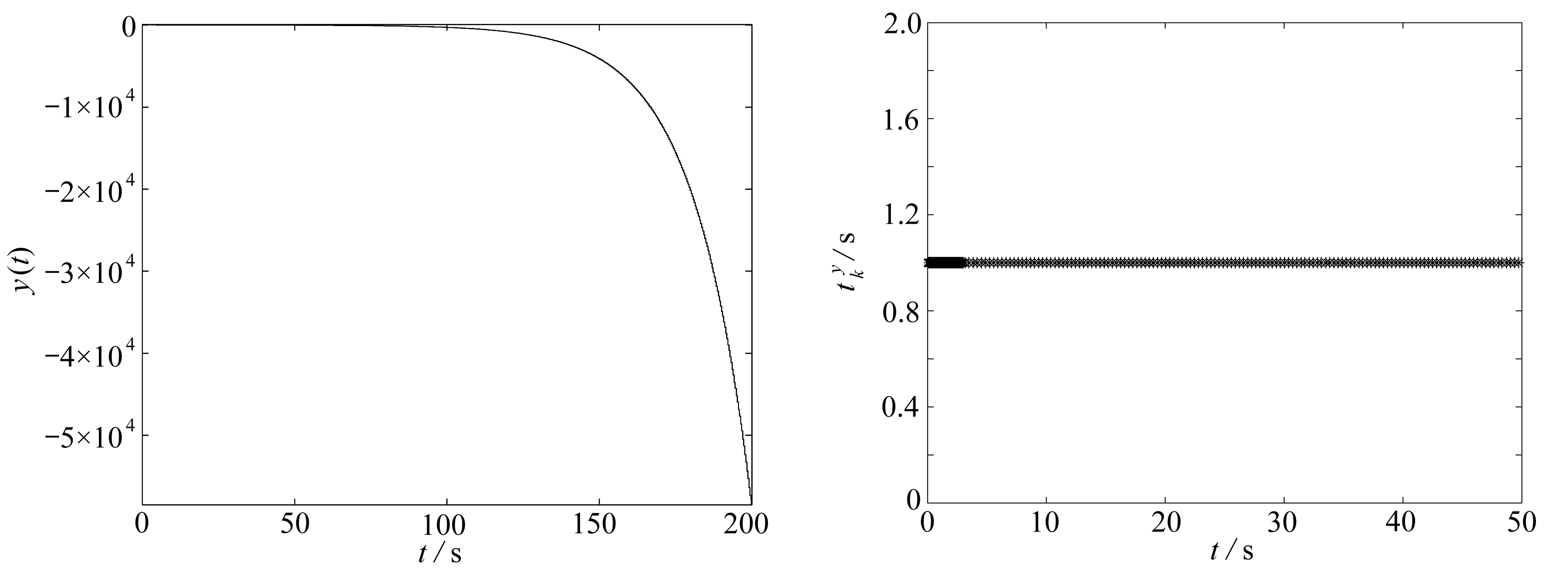Fig. 2 The network measurement output y(t) Fig. 3 The event trigger time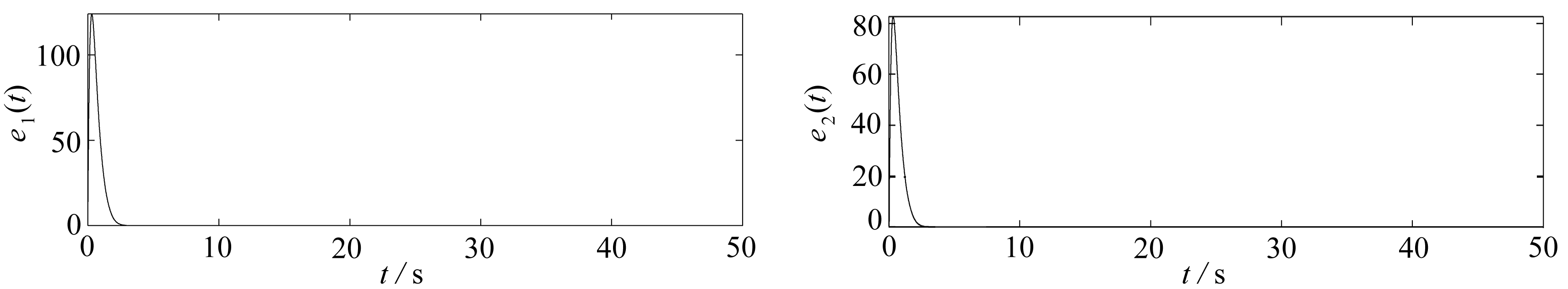(a) e1(t)(b) e2(t)

Fig. 4 The state trajectory of the error system

## 4 总 结

 WANG L, SONG Q, ZHAO Z, et al. Synchronization of two nonidentical complex-valued neural networks with leakage delay and time-varying delays[J]. Neurocomputing, 2019, 356: 52-59.

 WU H, FENG Y, TU Z, et al. Exponential synchronization of memristive neural networks with time delays[J]. Neurocomputing, 2018, 297: 1-7.

 舒含奇, 宋乾坤. 带有时滞的Clifford值神经网络的全局指数稳定性[J]. 应用数学和力学, 2017, 38(5): 513-525.(SHU Hanqi, SONG Qiankun. Global stability of Clifford-valued recurrent neural network with mixed time-varing delays[J]. Applied Mathematics and Mechanics, 2017, 38(5): 513-525.(in Chinese))

 张平奎, 杨绪君. 基于激励滑模控制的分数阶神经网络的修正投影同步研究[J]. 应用数学和力学, 2018, 39(3): 343-354.(ZHANG Pingkui, YANG Xujun. Modiffied projective synchronization of a class of fractional-order neural networks based on active sliding mode control[J]. Applied Mathematics and Mechanics, 2018, 39(3): 343-354.(in Chinese))

 闫欢, 赵振江, 宋乾坤. 具有泄漏时滞的复值神经网络的全局同步性[J]. 应用数学和力学, 2016, 37(8): 832-841.(YAN Huan, ZHAO Zhenjiang, SONG Qiankun. Global synchronization of complex-valued neural network with leakage time delays[J]. Applied Mathematics and Mechanics, 2016, 37(8): 832-841.(in Chinese))

 SHAO H, LI H, ZHU C. New stability results for delayed neural networks[J]. Applied Mathematics and Computation, 2017, 311: 324-334.

 FUAD E, LUO Y, LIU Y, et al. State estimation for delayed neural networks with stochastic communication protocol: the finite-time case[J]. Neurocomputing, 2018, 281: 86-95.

 ARPIT B, ARUNA T, HARSHIT B, et al. A genetically optimized neural network model for multi-class classification[J]. Expert Systems With Applications, 2016, 60: 211-221.

 GABRIEL V, JUAN F D P, PABLO C, et al. Artificial neural networks used in optimization problems[J]. Neurcomputing, 2018, 272: 10-16.

 MARAT A, MEHMET O. Generation of cyclic/toroidal chaos by Hopfield neural networks[J]. Neurcomputing, 2014, 145: 230-239.

 YANG X, YUAN Q. Chaos and transient chaos in simple Hopfield neural networks[J]. Neurcomputing, 2005, 69(1): 232-241.

 CHEN Y, LIU Q, LU R, et al. Finite-time control of switched stochastic delayed systems[J]. Neurcomputing, 2016, 191: 374-379.

 LI X, YANG X, SONG S. Lyapunov conditions for finite-time stability of time-varying time-delay systems[J]. Automatica, 2019, 103: 135-140.

 HU J, SUI G. Fixed-time control of static impulsive neural networks with infinite distributed delay and uncertainty[J]. Communications in Nonlinear Science and Numerical Simulation, 2019, 78: 104848.

 ZHOU J, ZHAO T. State estimation for neural networks with two additive time-varying delay components using delay-product-type augmented Lyapunov-Krasovskii functionals[J]. Neurocomputing, 2019, 350: 155-169.

 LIU Y, SHEN B, LI Q. State estimation for neural networks with Markov-based nonuniform sampling: the partly unknown transition probability case[J]. Neurocomputing, 2019, 357: 261-270.

 LI Q, ZHU Q, ZHONG S, et al. State estimation for uncertain Markovian jump neural networks with mixed delays[J]. Neurocomputing, 2016, 182: 82-93.

 SYED A M, SARAVANAN S, ARIK S. Finite-time H state estimation for switched neural networks with time-varying delays[J]. Neurocomputing, 2016, 207: 580-589.

 TAE H, JU H, HOYOUL J. Network-based H state estimation for neural networks using imperfect measurement[J]. Applied Mathematics and Computation, 2018, 316: 205-214.

 DONG H, WANG Z, SHEN B, et al. Variance-constrained H control for a class of nonlinear stochastic discrete time-varying systems: the event-triggered design[J]. Automatica, 2016, 72: 28-36.

 LIU Y, WANG Z, HE X, et al. Event-triggered least squares fault estimation with stochastic nonlinearities[J]. IFAC Proceedings Volumes, 2014, 47(3): 1855-1860.

 XIE Y, LIN Z. Event-triggered global stabilization of general linear systems with bounded controls[J]. Automatica, 2019, 107: 241-254.

 SUN Y, YANG G. Event-triggered state estimation for networked control systems with lossy network communication[J]. Information Sciences, 2019, 492: 1-12.

 LIU D, YANG G. Robust event-triggered control for networked control systems[J]. Information Sciences, 2018, 459: 168-197.

 WANG Z, HU J, MA L. Event-based distributed information fusion over sensor networks[J]. Information Fusion, 2018, 39: 53-55.

 YU H, HE Y, WU M. Delay-dependent state estimation for neural networks with time-varying delay[J]. Neurocomputing, 2018, 275: 881-887.

 WANG Z, LIU Y, LIU X. State estimation for jumping recurrent neural networks with discrete and distributed delays[J]. Neural Networks, 2009, 22(1): 41-48.

 ZHANG W, WANG Z, LIU Y, et al. Event-based state estimation for a class of complex networks with time-varying delays: a comparison principle approach[J]. Physics Letters A, 2017, 381(1): 10-18.

 YANG W, LEI L, YANG C. Event-based distributed state estimation under deception attack[J]. Neurocomputing, 2017, 270: 145-151.

 SHI D, CHEN T, MOHAMED D. Event-based state estimation of linear dynamic systems with unknown exogenous inputs[J]. Automatica, 2016, 69: 275-288.

 GUAN Z, DAVID J H, SHEN X. On hybrid impulsive and switching systems and application to nonlinear control[J]. IEEE Transactions on Automatic Control, 2005, 50(7): 1058-1062.

 BOYD S, EL GHAOUI L, FERON E, et al. Linear Matrix Inequalities in System and Control Theory[M]. Society for Industrial and Applied Mathematics, 1994.

(Contributed by SONG Qiankun, M. AMM Editorial Board)

# Event-BasedStateEstimationforNeuralNetworkWithTime-VaryingDelayandInfinite-DistributedDelay

DU Yuwei, LI Bing, SONG Qiankun

(College of Mathematics and Statistics, Chongqing Jiaotong University,Chongqing 400074, P.R.China)

Abstract: The event-based state estimation problem was investigated for a class of neural networks with mixed delays. A novel event-triggering scheme depending on both the output and exponential decay function was designed to reduce the frequency of updating. In view of both the mixed delays and the event-triggering properties, a new state estimation error system was built. The exponential stability of the error system was derived with the Lyapunov function and the inequality technique. The Zeno phenomenon was analyzed and excluded. Finally, a numerical example and its simulations were presented to illustrate the effectiveness of the proposed approach.

Key words: mixed delay; state estimation; event-triggering control; Zeno behavior; Lyapunov function

*收稿日期： 2019-12-13；

DOI: 10.21656/1000-0887.400377

Foundation item: The National Natural Science Foundation of China(61773004)